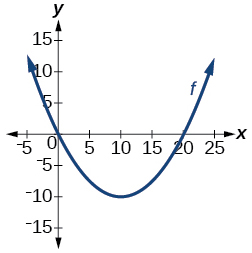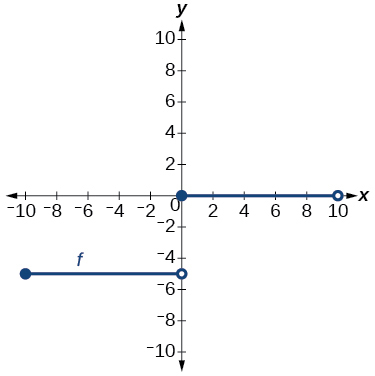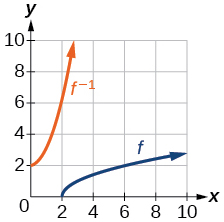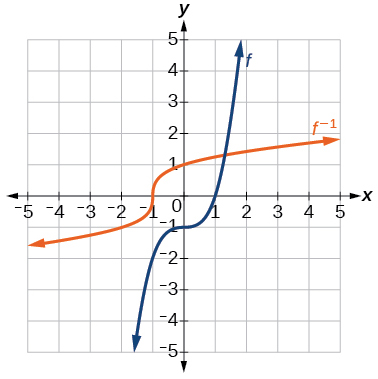# 3.7 Inverse functions  (Page 7/9)

 Page 7 / 9

For the following exercises, use function composition to verify that $\text{\hspace{0.17em}}f\left(x\right)\text{\hspace{0.17em}}$ and $\text{\hspace{0.17em}}g\left(x\right)\text{\hspace{0.17em}}$ are inverse functions.

$f\left(x\right)=\sqrt{x-1}\text{\hspace{0.17em}}$ and $\text{\hspace{0.17em}}g\left(x\right)={x}^{3}+1$

$f\left(x\right)=-3x+5\text{\hspace{0.17em}}$ and $\text{\hspace{0.17em}}g\left(x\right)=\frac{x-5}{-3}$

## Graphical

For the following exercises, use a graphing utility to determine whether each function is one-to-one.

$f\left(x\right)=\sqrt{x}$

one-to-one

$f\left(x\right)=\sqrt{3x+1}$

$f\left(x\right)=-5x+1$

one-to-one

$f\left(x\right)={x}^{3}-27$

For the following exercises, determine whether the graph represents a one-to-one function.not one-to-oneFor the following exercises, use the graph of $\text{\hspace{0.17em}}f\text{\hspace{0.17em}}$ shown in [link] .

Find $\text{\hspace{0.17em}}f\left(0\right).$

$3$

Solve $\text{\hspace{0.17em}}f\left(x\right)=0.$

Find $\text{\hspace{0.17em}}{f}^{-1}\left(0\right).$

$2$

Solve $\text{\hspace{0.17em}}{f}^{-1}\left(x\right)=0.$

For the following exercises, use the graph of the one-to-one function shown in [link] .

Sketch the graph of $\text{\hspace{0.17em}}{f}^{-1}.\text{\hspace{0.17em}}$Find

If the complete graph of $\text{\hspace{0.17em}}f\text{\hspace{0.17em}}$ is shown, find the domain of $\text{\hspace{0.17em}}f.\text{\hspace{0.17em}}$

$\left[2,10\right]$

If the complete graph of $\text{\hspace{0.17em}}f\text{\hspace{0.17em}}$ is shown, find the range of $\text{\hspace{0.17em}}f.$

## Numeric

For the following exercises, evaluate or solve, assuming that the function $\text{\hspace{0.17em}}f\text{\hspace{0.17em}}$ is one-to-one.

If $\text{\hspace{0.17em}}f\left(6\right)=7,\text{\hspace{0.17em}}$ find $\text{\hspace{0.17em}}\text{\hspace{0.17em}}{f}^{-1}\left(7\right).$

$6$

If $\text{\hspace{0.17em}}f\left(3\right)=2,\text{\hspace{0.17em}}$ find $\text{\hspace{0.17em}}{f}^{-1}\left(2\right).$

If $\text{\hspace{0.17em}}{f}^{-1}\left(-4\right)=-8,\text{\hspace{0.17em}}$ find $\text{\hspace{0.17em}}f\left(-8\right).$

$-4$

If $\text{\hspace{0.17em}}{f}^{-1}\left(-2\right)=-1,\text{\hspace{0.17em}}$ find $\text{\hspace{0.17em}}f\left(-1\right).$

For the following exercises, use the values listed in [link] to evaluate or solve.

$x$ $f\left(x\right)$
0 8
1 0
2 7
3 4
4 2
5 6
6 5
7 3
8 9
9 1

Find $\text{\hspace{0.17em}}f\left(1\right).$

$0$

Solve $\text{\hspace{0.17em}}f\left(x\right)=3.$

Find $\text{\hspace{0.17em}}{f}^{-1}\left(0\right).$

$\text{\hspace{0.17em}}1\text{\hspace{0.17em}}$

Solve $\text{\hspace{0.17em}}{f}^{-1}\left(x\right)=7.$

Use the tabular representation of $\text{\hspace{0.17em}}f\text{\hspace{0.17em}}$ in [link] to create a table for $\text{\hspace{0.17em}}{f}^{-1}\left(x\right).$

 $x$ 3 6 9 13 14 $f\left(x\right)$ 1 4 7 12 16
 $x$ 1 4 7 12 16 ${f}^{-1}\left(x\right)$ 3 6 9 13 14

## Technology

For the following exercises, find the inverse function. Then, graph the function and its inverse.

$f\left(x\right)=\frac{3}{x-2}$

$f\left(x\right)={x}^{3}-1$

${f}^{-1}\left(x\right)={\left(1+x\right)}^{1/3}$Find the inverse function of $\text{\hspace{0.17em}}f\left(x\right)=\frac{1}{x-1}.\text{\hspace{0.17em}}$ Use a graphing utility to find its domain and range. Write the domain and range in interval notation.

## Real-world applications

To convert from $\text{\hspace{0.17em}}x\text{\hspace{0.17em}}$ degrees Celsius to $\text{\hspace{0.17em}}y\text{\hspace{0.17em}}$ degrees Fahrenheit, we use the formula $\text{\hspace{0.17em}}f\left(x\right)=\frac{9}{5}x+32.\text{\hspace{0.17em}}$ Find the inverse function, if it exists, and explain its meaning.

${f}^{-1}\left(x\right)=\frac{5}{9}\left(x-32\right).\text{\hspace{0.17em}}$ Given the Fahrenheit temperature, $\text{\hspace{0.17em}}x,\text{\hspace{0.17em}}$ this formula allows you to calculate the Celsius temperature.

The circumference $\text{\hspace{0.17em}}C\text{\hspace{0.17em}}$ of a circle is a function of its radius given by $\text{\hspace{0.17em}}C\left(r\right)=2\pi r.\text{\hspace{0.17em}}$ Express the radius of a circle as a function of its circumference. Call this function $\text{\hspace{0.17em}}r\left(C\right).\text{\hspace{0.17em}}$ Find $\text{\hspace{0.17em}}r\left(36\pi \right)\text{\hspace{0.17em}}$ and interpret its meaning.

A car travels at a constant speed of 50 miles per hour. The distance the car travels in miles is a function of time, $\text{\hspace{0.17em}}t,\text{\hspace{0.17em}}$ in hours given by $\text{\hspace{0.17em}}d\left(t\right)=50t.\text{\hspace{0.17em}}$ Find the inverse function by expressing the time of travel in terms of the distance traveled. Call this function $\text{\hspace{0.17em}}t\left(d\right).\text{\hspace{0.17em}}$ Find $\text{\hspace{0.17em}}t\left(180\right)\text{\hspace{0.17em}}$ and interpret its meaning.

$t\left(d\right)=\frac{d}{50},\text{\hspace{0.17em}}$ $t\left(180\right)=\frac{180}{50}.\text{\hspace{0.17em}}$ The time for the car to travel 180 miles is 3.6 hours.

## Functions and Function Notation

For the following exercises, determine whether the relation is a function.

$\left\{\left(a,b\right),\left(c,d\right),\left(e,d\right)\right\}$

function

$\left\{\left(5,2\right),\left(6,1\right),\left(6,2\right),\left(4,8\right)\right\}$

${y}^{2}+4=x,\text{\hspace{0.17em}}$ for $\text{\hspace{0.17em}}x\text{\hspace{0.17em}}$ the independent variable and $\text{\hspace{0.17em}}y\text{\hspace{0.17em}}$ the dependent variable

not a function

Is the graph in [link] a function?

For the following exercises, evaluate the function at the indicated values: $\text{\hspace{0.17em}}\text{\hspace{0.17em}}\text{\hspace{0.17em}}f\left(-3\right);\text{\hspace{0.17em}}\text{\hspace{0.17em}}f\left(2\right);\text{\hspace{0.17em}}\text{\hspace{0.17em}}\text{\hspace{0.17em}}f\left(-a\right);\text{\hspace{0.17em}}\text{\hspace{0.17em}}\text{\hspace{0.17em}}-f\left(a\right);\text{\hspace{0.17em}}\text{\hspace{0.17em}}\text{\hspace{0.17em}}f\left(a+h\right).$

$f\left(x\right)=-2{x}^{2}+3x$

$f\left(-3\right)=-27;$ $f\left(2\right)=-2;$ $f\left(-a\right)=-2{a}^{2}-3a;$
$-f\left(a\right)=2{a}^{2}-3a;$ $f\left(a+h\right)=-2{a}^{2}+3a-4ah+3h-2{h}^{2}$

$f\left(x\right)=2|3x-1|$

For the following exercises, determine whether the functions are one-to-one.

what are you up to?
nothing up todat yet
Miranda
hi
jai
hello
jai
Miranda Drice
jai
aap konsi country se ho
jai
which language is that
Miranda
I am living in india
jai
good
Miranda
what is the formula for calculating algebraic
I think the formula for calculating algebraic is the statement of the equality of two expression stimulate by a set of addition, multiplication, soustraction, division, raising to a power and extraction of Root. U believe by having those in the equation you will be in measure to calculate it
Miranda
state and prove Cayley hamilton therom
hello
Propessor
hi
Miranda
the Cayley hamilton Theorem state if A is a square matrix and if f(x) is its characterics polynomial then f(x)=0 in another ways evey square matrix is a root of its chatacteristics polynomial.
Miranda
hi
jai
hi Miranda
jai
thanks
Propessor
welcome
jai
What is algebra
algebra is a branch of the mathematics to calculate expressions follow.
Miranda
Miranda Drice would you mind teaching me mathematics? I think you are really good at math. I'm not good at it. In fact I hate it. 😅😅😅
Jeffrey
lolll who told you I'm good at it
Miranda
something seems to wispher me to my ear that u are good at it. lol
Jeffrey
lolllll if you say so
Miranda
but seriously, Im really bad at math. And I hate it. But you see, I downloaded this app two months ago hoping to master it.
Jeffrey
which grade are you in though
Miranda
oh woww I understand
Miranda
Jeffrey
Jeffrey
Miranda
how come you finished in college and you don't like math though
Miranda
gotta practice, holmie
Steve
if you never use it you won't be able to appreciate it
Steve
I don't know why. But Im trying to like it.
Jeffrey
yes steve. you're right
Jeffrey
so you better
Miranda
what is the solution of the given equation?
which equation
Miranda
I dont know. lol
Jeffrey
Miranda
Jeffrey
answer and questions in exercise 11.2 sums
how do u calculate inequality of irrational number?
Alaba
give me an example
Chris
and I will walk you through it
Chris
cos (-z)= cos z .
cos(- z)=cos z
Mustafa
what is a algebra
(x+x)3=?
6x
Obed
what is the identity of 1-cos²5x equal to?
__john __05
Kishu
Hi
Abdel
hi
Ye
hi
Nokwanda
C'est comment
Abdel
Hi
Amanda
hello
SORIE
Hiiii
Chinni
hello
Ranjay
hi
ANSHU
hiiii
Chinni
h r u friends
Chinni
yes
Hassan
so is their any Genius in mathematics here let chat guys and get to know each other's
SORIE
I speak French
Abdel
okay no problem since we gather here and get to know each other
SORIE
hi im stupid at math and just wanna join here
Yaona
lol nahhh none of us here are stupid it's just that we have Fast, Medium, and slow learner bro but we all going to work things out together
SORIE
it's 12
what is the function of sine with respect of cosine , graphically
tangent bruh
Steve
cosx.cos2x.cos4x.cos8x
sinx sin2x is linearly dependent
what is a reciprocal
The reciprocal of a number is 1 divided by a number. eg the reciprocal of 10 is 1/10 which is 0.1
Shemmy
Reciprocal is a pair of numbers that, when multiplied together, equal to 1. Example; the reciprocal of 3 is ⅓, because 3 multiplied by ⅓ is equal to 1
Jeza
each term in a sequence below is five times the previous term what is the eighth term in the sequence
I don't understand how radicals works pls
How look for the general solution of a trig functionByByBy Szilárd JankóBy Madison ChristianBy John GabrieliBy OpenStaxBy Cameron CaseyBy Wey HeyBy Cath YuBy Brooke DelaneyBy Miranda ReisingBy Brooke Delaney